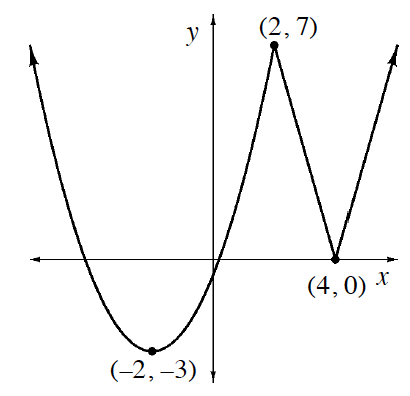### Home > APCALC > Chapter 1 > Lesson 1.4.3 > Problem1-170

1-170.

Use a quadratic function and an absolute value function to write a piecewise-defined function that will produce the graph shown below.Vertex form of a parabola: $y=a(x−h)^2+k$, where $(h,k)$ is the vertex. Absolute value: $y=a|x−h|+k$.

Solve for $a$ by evaluating both equations at $(2,7)$.

Because the function for this graph is piecewise, there is an $x$-coordinate / boundary point where each function ceases to defined.